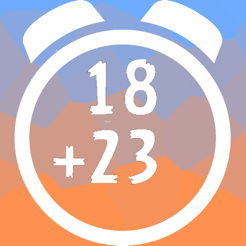## Screenshots

•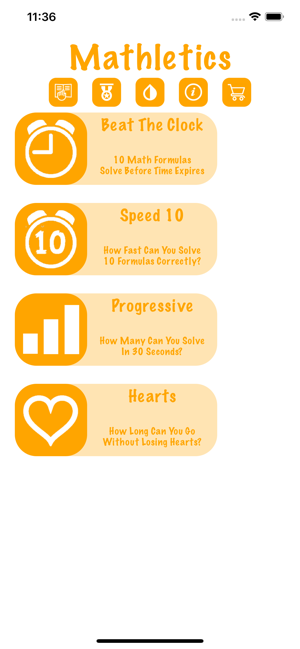•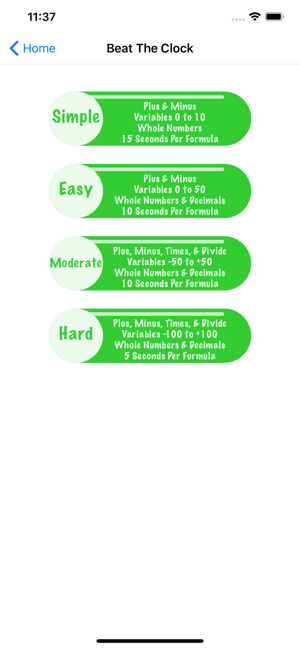•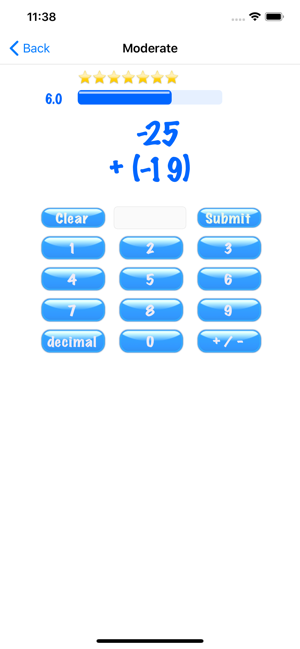•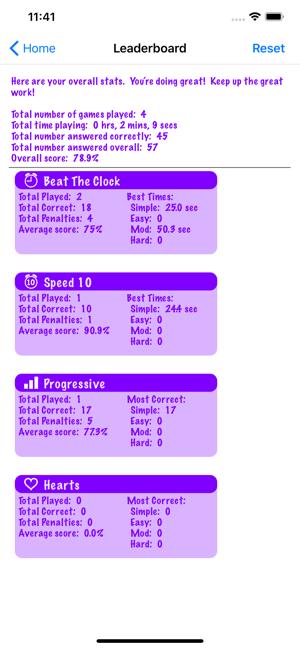## Description

Whether you love math, need to work on your math, or just have a couple of minutes to spare, Mathletics Speed Math is the app for you! Mathletics Speed Math is a fun app to help improve upon your math skills! Show your brain some love by answering an unlimited number of formulas in a short amount of time. How quickly can you answer all of the formulas in a set?

Mathletics Speed Math contains 4 different game modes:
* Beat The Clock: Solve 10 formulas before time expires on each
* Speed 10: Solve 10 formulas as quickly as you can
* Progressive: Answer as many formulas as you can in 30 seconds
* Hearts: Solve as many formulas as you can without losing all of your hearts

Each game mode has 4 levels of difficulty:
* Simple: Addition and subtraction formulas with whole numbers ranging from 0 to 10
* Easy: Addition and subtraction formulas with whole numbers and decimals ranging from 0 to 50
* Moderate: Addition, subtraction, multiplication, and division formulas with whole numbers and decimals ranging from -50 to +50
* Hard: Addition, subtraction, multiplication, and division formulas with whole numbers and decimals ranging from -100 to +100

Free users may enjoy unlimited access to Beat The Clock and Speed 10 game modes in levels Simple, Easy, and Moderate.

## Information

Seller
Visual Veggies
Size
7.3 MB
Category
Games
Compatibility

Languages

English

Age Rating
4+
Price
Free
In-App Purchases
•